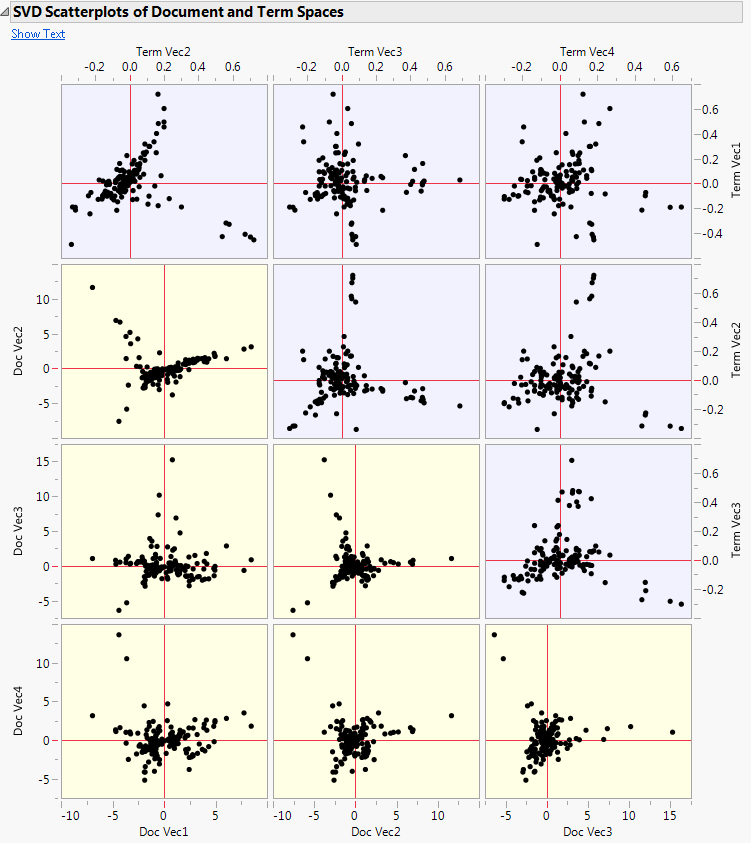Publication date: 08/13/2020

##SVD Report Options

The SVD red triangle menu contains the following options:

SVD Scatterplot Matrix

Shows or hides a scatterplot matrix of the term and document singular value decomposition vectors. You are prompted to select the size of the scatterplot matrix when you select this option. This scatterplot matrix enables you to visualize more than the first two dimensions of the singular value decomposition. The Show Text button opens a window that contains the text of the selected documents.

Figure 12.11 SVD Scatterplots of Document and Term SpacesTopic Analysis, Rotated SVD

Performs a varimax rotated partial singular value decomposition of the document term matrix to produce groups of terms called topics. You can select this option multiple times to find different numbers of topics. See Topic Analysis.

Cluster Terms

Shows or hides a hierarchical clustering analysis of the terms in the data. To the right of the dendrogram, there are options to set the number of clusters and save the clusters to a data table. For each term, this data table contains its frequency, the number of documents that contain it, and its assigned cluster. For more information about hierarchical clustering and dendrograms, see Hierarchical Cluster in Multivariate Methods.

Cluster Documents

Shows or hides a hierarchical clustering analysis of the documents in the data. To the right of the dendrogram, there are options to do the following: set the number of clusters, save the clusters to a column in the data table, and show the documents in a selected branch of the dendrogram plot.

Save Document Singular Vectors

Saves a user-specified number of singular vectors from the document singular value decomposition as columns to the data table. The first two saved columns represent the points plotted in the document SVD plot. See Latent Semantic Analysis (SVD).

Save Singular Vector Formula

Saves a formula column with the Vector modeling type that contains the document singular value decomposition to the data table. The resulting column uses the Text Score() JSL function. For more information about this function, see Help > Scripting Index.

Save Term Singular Vectors

Saves a user-specified number of singular vectors from the terms singular value decomposition as columns to a new data table where each row corresponds to a term. If a Term Table data table is already open, this option saves the columns to that data table. The first two saved columns represent the points plotted in the term SVD plot. See Latent Semantic Analysis (SVD).

Remove

Removes the SVD report from the Text Explorer report window.